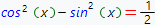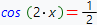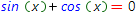# Trigonometric equations online calculatorThe general form of trigonometric equation is

f(trig(x)) = 0

where f - some arbitrary function, trig(x) - some trigonometric function.

As a rule, to solve trigonometric equation one need to transform it to the simplier form which has a known solution. The transformation can be done by using different trigonometric formulas.

For example, consider the solution of trigonometric equationBy using the cosine double angle formula, transform this equation to the simplier one:The received equation is the very simple and has known solution. Our online calculator, build on Wolfram Alpha system is able to solve more complex trigonometric equations with step by step solution.

Trig equations solverTrigonometric equation you want to solve: